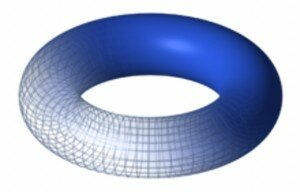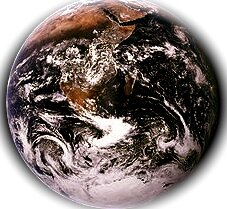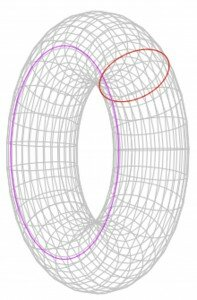# Torus Math – The DoughnutWelcome Back to Math Class!  We trust all had a wonder-filled summer filled with fun spherical geometric shapes and bursting color!  Our Back-to-school math topic this autumn is about the math magic of the Torus. A torus is: the area and volume of, basically, a doughnut shape, shown here:
http://en.wikipedia.org/wiki/File:Torus.png
The author of harmonic resolution; The Portacle, describes that the torus’ special form has been used to describe and/or represent a number of things in our “real” actual material world, as well as, our “imaginary” potential one; and is defined by two parameters: the radius of the torus (that is, the radius of the torus’s defining circle, measured from the origin) and the radius of the tube (the perpendicular distance from the defining circle to the surface of the torus). These are R and r, respectively (R > r).   -In this link, there is a Great fluid-moving image of a spinning torus, about 4/5th down the page.)  Wikipedia has a diagram of the torus as being the product of 2 circles, one sideways inside the other: See image to the left here: A torus is the product of two circles, in this case the red circle is swept around the axis defining the pink circle. R is the radius of the pink circle, r is the radius of the red one; Image drawn August 28, 2014: http://en.wikipedia.org/wiki/File:Torus_cycles.svgSome say that Earth is neither flat or round, but spherical, as in that of a doughnut toroid or even an apple, curving down and curving upward at the North and South poles.  One image we find most interesting is just under the blue torus image, and it is a mutable image of a ring torus turning itself into a horn torus, and this is one of the images that mathematicians today are calculating that Earth is most closer to as a realistic geometric shape, than was once thought.  Earth, in this respect is today referred to as the tube torus, a shape that occurs often in Nature.  If you click on the link, you can see just to the right under the blue torus, a better mental picture of our reference: As the distance to the axis of revolution decreases, the ring torus becomes a horn torus, then a spindle torus, and finally degenerates into a sphere; www.wikipedia.org/wiki/torus.http://en.wikipedia.org/wiki/File:Torus.png
(There are several on-line sources of the manifolds, 3 of which quote the same information:  Joyce, Wikipedia and Harmonic Resolution.com, all denoting that manifolds are today, a major branch of geometric study, a curved space of some dimension. For example, the surface of a sphere, and the torus (the surface of a doughnut), are both 2-dimensional flat-surface – perceived manifolds. The manifold itself is the background for some mathematical object defined upon it, as a canvas is the background for an oil painting. This kind of geometry, although very abstract, is closer to the real world than you might think. Einstein’s theory of General Relativity describes the Universe – the whole of space and time – as a 4-dimensional manifold.  Wikipedia has wonderful moving images of the manifold turning into the torus and back into a manifold flat surface again, and is worth having a gander for a more picturesque idea of what these terms are in Math, under the subtitle “ Flat Torus“: http://en.wikipedia.org/wiki/Torus: http://en.wikipedia.org/wiki/File:Torus_from_rectangle.gif

Dominic Joyce, Universty of Oxford, (www. maths.ox. ac.uk/people/profiles/dominic.joyce) iterates that today’s popular geometric math is in manifolds, no longer Euclid’s triangles and circles, and says that popularity went out with the Arc!  We think he is referring to Noah’s Arc, which many would probably disagree about Noah Arc’s popularity because of all the beautyful animals on the Arc!  But that is digressing….Joyce says that, Space itself is not flat, but curved. The curvature of space is responsible for gravity….and that, Everything in the universe – light, subatomic particles, pizzas, and even, yourself – is described in terms of a geometrical structure on the space-time 4-manifold.  Joyce says that, according to some physicists, the universe is now considered to be a 12 (or 13, 15, or 17, by some!) dimensional nested manifold.

In “superstring” physics, the torus is known as the “perfect” shape…but String Theory we can discuss on another day!

For more fun and interesting Learning Math Games, you can visit us here:

http://www.math-lessons.ca/activities/Geometry.html

http://www.math-lessons.ca/activities/FractionsBoard5.html

http://www.math-lessons.ca/timestables/times-tables.html

http://www.math-lessons.ca/activities/FractionsBoard4.html